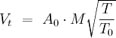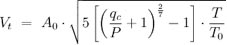# Glossary of Aviation Terms | True Airspeed

## True Airspeed | Paramount Business Jets

True airspeed is the speed of an aircraft moving through the air. As an airplane moves through the atmosphere, the air passes under the wings at a given speed. The wind moving with or against the aircraft directly affects the speed of the air moving under the wings, and thus directly affects the true airspeed. If there was no wind, then the true airspeed of the aircraft would be equal to the ground speed of the aircraft. So, in a situation where wind is present, the velocity of the wind must be taken from the ground speed of the plane, and this is best represented by the equation:

Vt = Vg - Vw

where: Vt = true airspeed, Vg = ground speed, Vw = wind speed

As well as wind, temperature, and altitude also impact true airspeed. When altitude or air temperature increases, the density of air decreases and the true airspeed increases. This is because there is less air to put up resistance against the aircraft moving forward, so the aircraft moves faster through the air.

Pilots use true airspeed for a number of calculations, so it is important to understand that in the cockpit, true airspeed isn’t calculated using wind velocity. Instead, true airspeed is calculated by correcting calibrated airspeed for non-standard temperature and pressure altitude. If you were flying at sea level conditions, true airspeed would be equal to calibrated airspeed and there would be no need for a calculation. Since the majority of the time, planes fly at high altitudes, an equation is needed to calculate true airspeed, and this is best done using your mach number (M) with the equation:where: Ao = speed of sound at standard sea level, M = Mach number, T = temperature (kelvins), To = standard temperature at sea level (kelvins)

If you do not know your Mach number, then you can substitute it with your pressure using the equation:where: Ao = speed of sound at sea lever, qc = impact pressure, P = static pressure, T = temperature (K), To = standard sea lever temperature.

Pilots also use true airspeed when performing dead reckoning or pilotage. They also use true airspeed when filing VFR or IFR flight plans with a weather briefer or when figuring out distance times and wind corrections.

## Get a Quote for Your trip

•••••••••